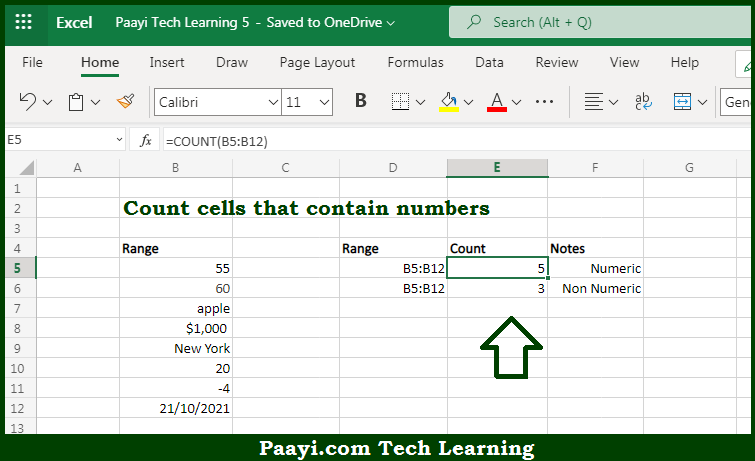# Learn How to COUNT Cells that Contain Numbers in Microsoft Excel

Written by | 0 Comments | 552 Views

In this article, you will learn how to COUNT various things in Microsoft Excel using a single or combination of functions and its purpose in Microsoft Excel. You will also get to know how to count cells that contain numbers and see the generic formula.

Count Cells that Contain Numbers in Microsoft Excel

The main purpose of this formula is to count the number of cells that contain numbers. Here we will learn how to count cells that contain numeric values.  That implies, with the help of a formula based on the  COUNT function you can able to count the number of cells that contain numbers. The generic form of the formula provided below "rng" represents a range of cells. So, with the help of this formula, you can able to count the number of cells that contain numbers.

General Formula to COUNT Cells that Contain Numbers

=COUNT(rng)

### The Explanation for the COUNT Cells that Contain NumbersSo we know that with the help of the given formula above you can able to count the number of cells that contain numbers. Here we will learn how to count cells that contain numeric values. As we know that, when you use the COUNTIF function is having fully automatic features. It counts the number of cells in the range that contains numbers and returns the result in the number form. It should be noted that o count the number of cells in a range not having any numbers, you can use this SUMPRODUCT function. So, with the help of this formula, you can able to count cells that contain numbers.